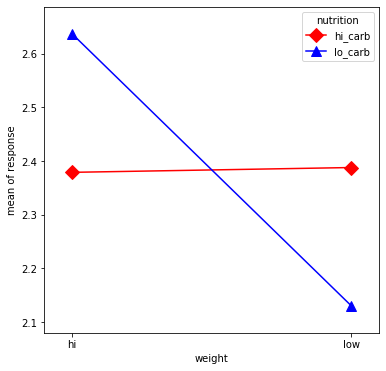Plot Interaction of Categorical Factors¶

In this example, we will visualize the interaction between categorical factors. First, we will create some categorical data are initialized. Then plotted using the interaction_plot function which internally re-codes the x-factor categories to integers.

In :
%matplotlib inline

import numpy as np
import matplotlib.pyplot as plt
import pandas as pd
from statsmodels.graphics.factorplots import interaction_plot
In :
np.random.seed(12345)
weight = pd.Series(np.repeat(['low', 'hi', 'low', 'hi'], 15), name='weight')
nutrition = pd.Series(np.repeat(['lo_carb', 'hi_carb'], 30), name='nutrition')
days = np.log(np.random.randint(1, 30, size=60))
In :
fig, ax = plt.subplots(figsize=(6, 6))
fig = interaction_plot(x=weight, trace=nutrition, response=days,
colors=['red', 'blue'], markers=['D', '^'], ms=10, ax=ax)